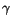Next: Space Travel Up: Other Implications of Special Previous: Other Implications of Special

## E = mc2

One thing you will notice about the procedure defined at the end of the previous Section 11.5 is that the gain when you try to accelerate a ball to the speed of light in steps, the increase in velocity relative to the initial frame gets less and less, each time you repeat the process. The faster the ball is going, the hard it is to accelerate further. Now recall the definition of inertial mass: it is the measure of an object's resistance to a change in motion. In Newtonian physics inertial mass is a property of the object alone, and does not depend in any way on its state of motion. A one kilogram ball has a mass of one kilogram no matter how fast it happens to be moving. The situation is drastically different in Special Relativity. The faster a mass moves, the harder it is to accelerate. Hence its inertial mass depends on its speed! It will come as no surprise that this dependence is determined by the time dilation factor. In particular, if an object is moving relative to you at a speed of v, you will measure an inertial mass that increases by a factor ofcompare to its value m0 at rest:

 m =m0 (11.14)

m0 is called the rest mass of the object, for obvious reasons. For example, if an object is moving at .99c, its mass will appear to be a factor of seven times larger than its rest mass. Again, because of the form ofthis effect is negligible at velocities that are small compared to the speed of light. However, the increase of inertial mass with velocity has been measured in the large accelerators used by particle physicists to create fast moving beams of electrons. The faster the electrons are moving, the harder it is to further increase their kinetic energy.

There is another profound implication of the above considerations that Einstein was the first to realize. We know that when you increase the velocity of an object, you are giving it kinetic energy. We also know that kinetic energy can be converted into other forms of energy, such as potential energy and heat energy and vice versa. If increasing the velocity of an object increases its inertial mass, then in some sense we are converting kinetic energy into mass. Einstein reasoned that mass and energy must somehow be different manifestations of the same thing. In particular if we can convert kinetic energy into mass, we should also be able to convert mass into energy. The special theory of relativity in fact gives an explicit expression for how mass and energy are related. You have probably seen it before.

 E = mc2 = m0c2 (11.15)

Thus as expected from earlier objects, the energy of an object increase without bound as its velocity approaches the speed of light. The surprising aspect of 11.15 is that even when an object is at rest, it has energy. When v = 0,= 1 amd m = m0 so that for a mass at rest:

 E0 = m0c2 (11.16)

The energy of an object at rest is related to its mass by the factor c2 which is a very large number. The consequence is that very small masses can produce vast amounts of energy, and nuclear power plants and bombs are effective because they can convert mass into other forms of energy. In fact, if all the mass in a single grain of sand could be converted into energy, it could provide enough electricity to light up a whole city for a year. As we will see in the Section on Nuclear Physics, however, it is not easy to convert large amounts of mass into energy.Next: Space Travel Up: Other Implications of Special Previous: Other Implications of Special
modtech@theory.uwinnipeg.ca
1999-09-29